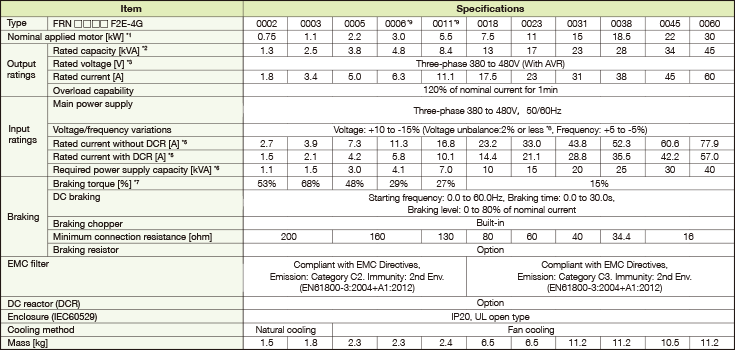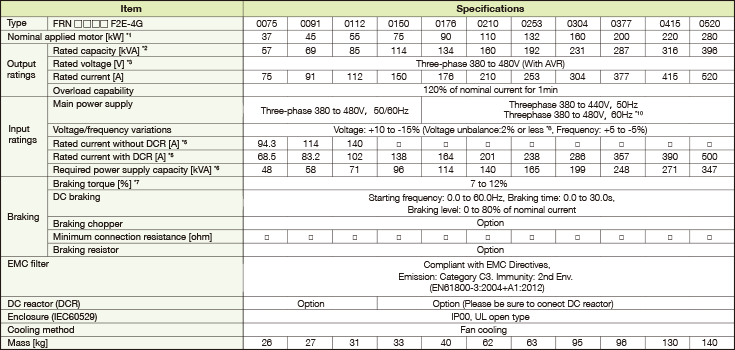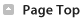## Specifications

## Standard Specifications

### Three phase 400V class series (EMC Filter Built-in Type)*1 When selecting the inverter rating for Fuji 4-pole standard motor, consider not only the rating capacity(kW) is enoughbut also inverter output current is larger than selected the motor's nominal current. Rated capacity is calculated by assuming the output rated voltage as 440 V. Output voltage cannot exceed the power supply voltage.Type 0002 to 0011：8kHz, type 0018 to 0045：10kHz, type 0060 to 0150：6kHz, type 0176 to 0520：4kHz When the carrier frequency (F26) is set to below value or higher, the inverter is sure to be necessary to derateits nominal current. The value is calculated assuming that the inverter is connected with a power supply with the capacity of 500 kVA(or 10 times the inverter capacity if the inverter capacity exceeds 50 kVA) and %X is 5%.Be sure to use the DCR when applicable motor capacity is 75kW or above. Obtained when a DC reactor (DCR) is used. Average braking torque for the motor running alone. (It varies with the efficiency of the motor.) Voltage unbalance (%) =(Max. voltage (V) - Min. voltage (V))/Three -phase average voltage (V) × 67 (IEC 61800 - 3)If this value is 2 to 3%, use an optional AC reactor (ACR). Type 0006 and 0011: allowable ambient temperature 40℃ (+104 °F) or less.The rated output current is decreased 1% for every 1 ℃ (1.8 °F) when ambient temperature is +40℃ (+104 °F) or more. The 400 V class series with type 0176 or above is equipped with a set of switching connectors (male) which should be configured according to the power source voltage and frequency.